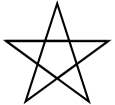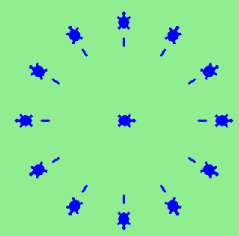# 4.11. Exercises¶

1. Write a program that prints We like Python's turtles! 100 times.

1. Turtle objects have methods and attributes. For example, a turtle has a position and when you move the turtle forward, the position changes. Think about the other methods shown in the summary above. Which attibutes, if any, does each method relate to? Does the method change the attribute?

1. Write a program that uses a for loop to print
One of the months of the year is January
One of the months of the year is February
One of the months of the year is March
etc …
1. Assume you have a list of numbers 12, 10, 32, 3, 66, 17, 42, 99, 20

1. Write a loop that prints each of the numbers on a new line.

2. Write a loop that prints each number and its square on a new line.

1. Use for loops to make a turtle draw these regular polygons (regular means all sides the same lengths, all angles the same):

• An equilateral triangle

• A square

• A hexagon (six sides)

• An octagon (eight sides)

# draw an equilateral triangle
import turtle

wn = turtle.Screen()
norvig = turtle.Turtle()

for i in range(3):
norvig.forward(100)

# the angle of each vertice of a regular polygon
# is 360 divided by the number of sides
norvig.left(360/3)

wn.exitonclick()

# draw a square
import turtle

wn = turtle.Screen()
kurzweil = turtle.Turtle()

for i in range(4):
kurzweil.forward(100)
kurzweil.left(360/4)

wn.exitonclick()

# draw a hexagon
import turtle

wn = turtle.Screen()
dijkstra = turtle.Turtle()

for i in range(6):
dijkstra.forward(100)
dijkstra.left(360/6)

wn.exitonclick()

# draw an octogon
import turtle

wn = turtle.Screen()
knuth = turtle.Turtle()

for i in range(8):
knuth.forward(75)
knuth.left(360/8)

wn.exitonclick()

1. Write a program that asks the user for the number of sides, the length of the side, the color, and the fill color of a regular polygon. The program should draw the polygon and then fill it in.

1. A drunk pirate makes a random turn and then takes 100 steps forward, makes another random turn, takes another 100 steps, turns another random amount, etc. A social science student records the angle of each turn before the next 100 steps are taken. Her experimental data is 160, -43, 270, -97, -43, 200, -940, 17, -86. (Positive angles are counter-clockwise.) Use a turtle to draw the path taken by our drunk friend. After the pirate is done walking, print the current heading.

1. On a piece of scratch paper, trace the following program and show the drawing. When you are done, press run and check your answer.

1. Write a program to draw a shape like this:1. Write a program to draw a face of a clock that looks something like this:1. Write a program to draw some kind of picture. Be creative and experiment with the turtle methods provided in Summary of Turtle Methods.